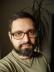## Stones and Jewels

Create a script that accepts two strings, let’s call them “stones” and “jewels”. It should print the count of “letters” from the string “stones” found in the string “jewels”. For example, if your stones is “chancellor” and “jewels” is “chocolate”, then the script should print 8. To keep it simple, only A-Z, a-z characters are acceptable. Also, make the comparison case sensitive.

The most important thing is to realise that we only want to consider unique characters in the “stones”. My initial idea was for each character of the “stones” to count how many times it appears in the “jewels”. Remember that the global matching operator `m//g` returns the number of matches in list context.

Let’s call the subroutine with two names parameters, stones and jewels, each of them containing a string.

``````use List::Util qw{ uniq };

sub count1 {
my %args = @_;
my \$count = 0;
\$count += () = \$args{jewels} =~ /\$_/g
for uniq(split //, \$args{stones});
return \$count
}``````

You probably already know the Saturn operator `=()=` (it also has a NSFW name “Goatse”). Here, we use its variant `+=()=` which is like the Voyager probe during its encounter with Saturn.

But running a regex match tends to be slow compared to some easier operations like hash lookup. Instead of matching each character, we can store all the “stones” into a hash and only count which “jewels” exist in it.

``````sub count2 {
my %args = @_;
my \$count = 0;
my %stones;
undef @stones{ split //, \$args{stones} };
exists \$stones{\$_} and ++\$count
for split //, \$args{jewels};
return \$count
}``````

Unsurprisingly, this method is much faster than the first one (about 6 times faster on my machine and strings of length 30).

Nevertheless, we can modify the initial solution to be even faster. Instead of matching each character, let’s create a character class from the “stones”—we don’t even need to make them unique, as the regex engine will do it for us when compiling the regex.

``````sub count3 {
my %args = @_;
my \$count = 0;
\$count += () = \$args{jewels} =~ /[\$args{stones}]/g;
return \$count
}``````

It still uses the Voyager operator and is about 80% faster than the hash solution!

## Mean of Angles

Create a script that prints mean angles of the given list of angles in degrees.

The linked Wikipedia page shows a formula which can be coded directly (after we convert the angles from degrees to radians and back):

``````use List::Util qw{ sum };

use constant PI => 4 * atan2(1, 1);

sub mean {
my @radians = map \$_ * 2 * PI / 360, @_;
my \$x = 1 / @_ * sum(map sin, @radians);
my \$y = 1 / @_ * sum(map cos, @radians);
return atan2(1 / @_ * sum(map sin, @radians),
1 / @_ * sum(map cos, @radians))
/ 2 / PI * 360
}``````

It works almost correctly for the two examples given in the linked article (for the first one, a bit of rounding is needed):

``````use Test::More tests => 2;
is sprintf('%.3f', mean(0, 0, 90)), 26.565;
is mean(5, 15, 355), 5;``````

Unfortunately, it returns strange numbers when the angles are uniformly distributed on a circle. We need to check that both `\$x` and `\$y` aren’t too close to zero before returning any result. Let’s insert the following lines before the `return` line:

``````    die "No mean defined\n"
if \$x < 1e-15 && \$y < 1e-15;``````

And we can add a test for it, too:

``````use Test::Exception;
throws_ok { mean(0, 90, 180, 270) } qr/No mean defined/;``````I blog about Perl.